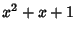## Irreducible Polynomial

A Polynomial or polynomial equation is said to be irreducible if it cannot be factored into polynomials of lower degree over the same Field.

The number of binary irreducible polynomials of degreeis equal to the number of-bead fixed Necklaces of two colors: 1, 2, 3, 4, 6, 8, 14, 20, 36, ... (Sloane's A000031), the first few of which are given in the following table.Polynomials 12,3,,4,,,